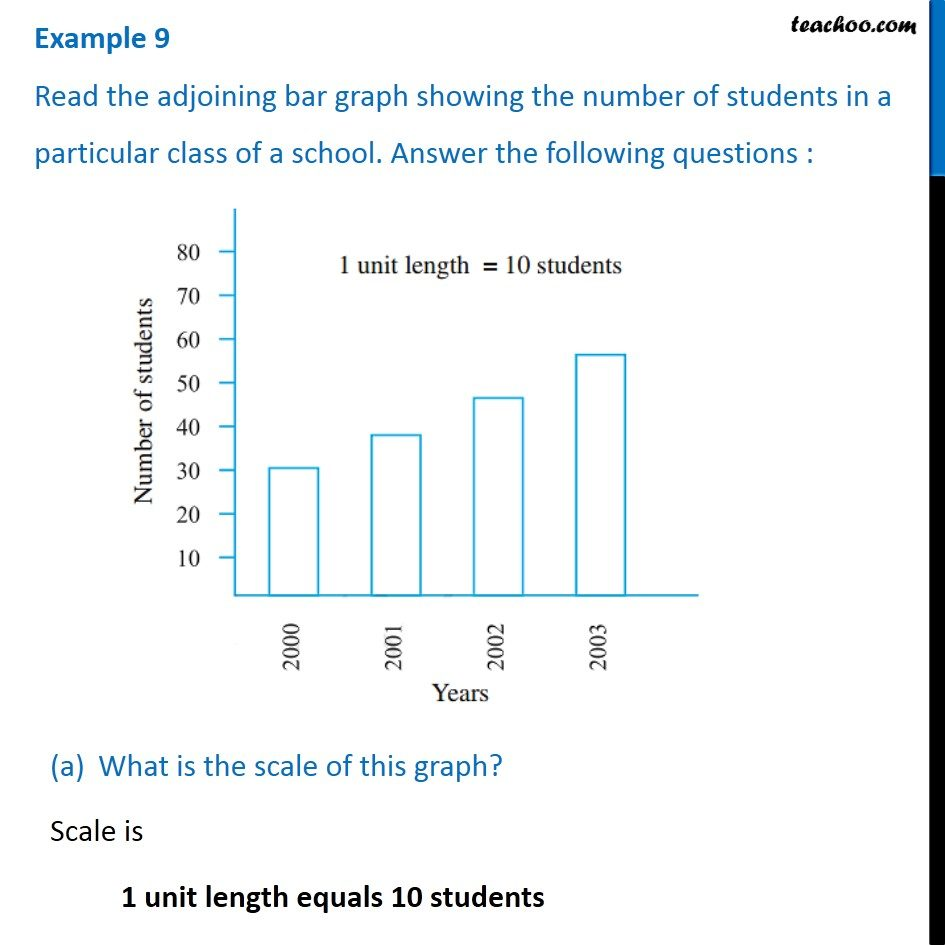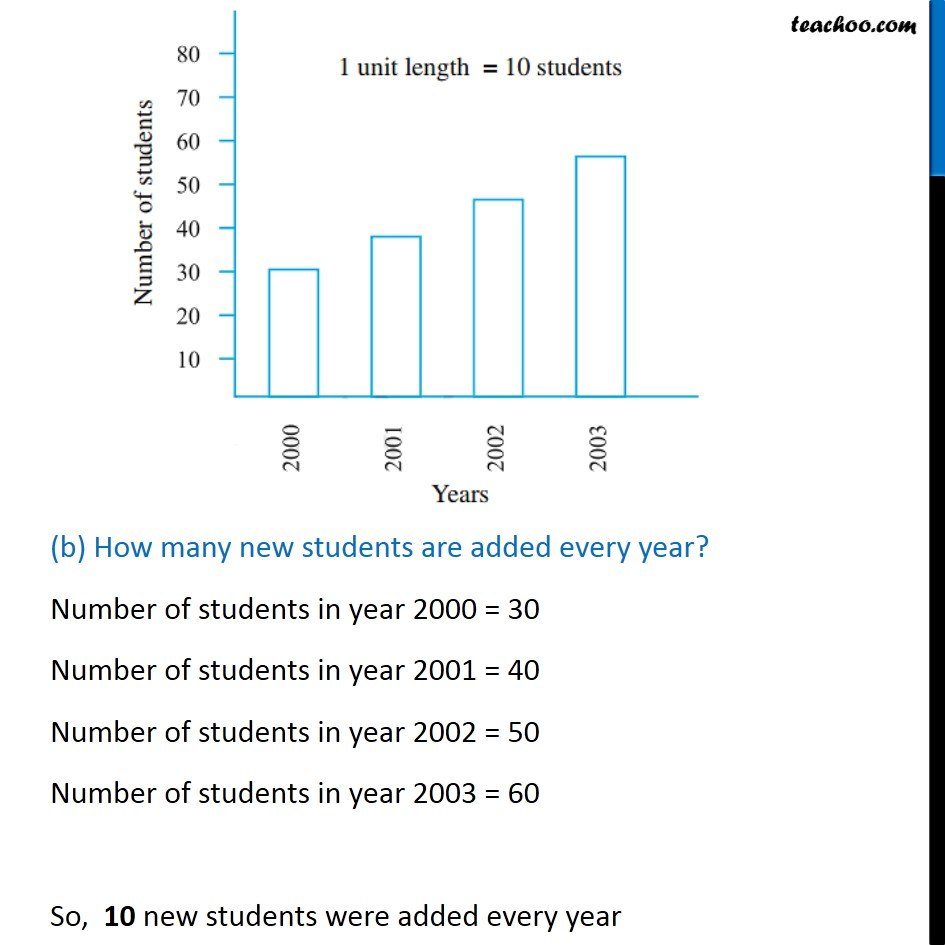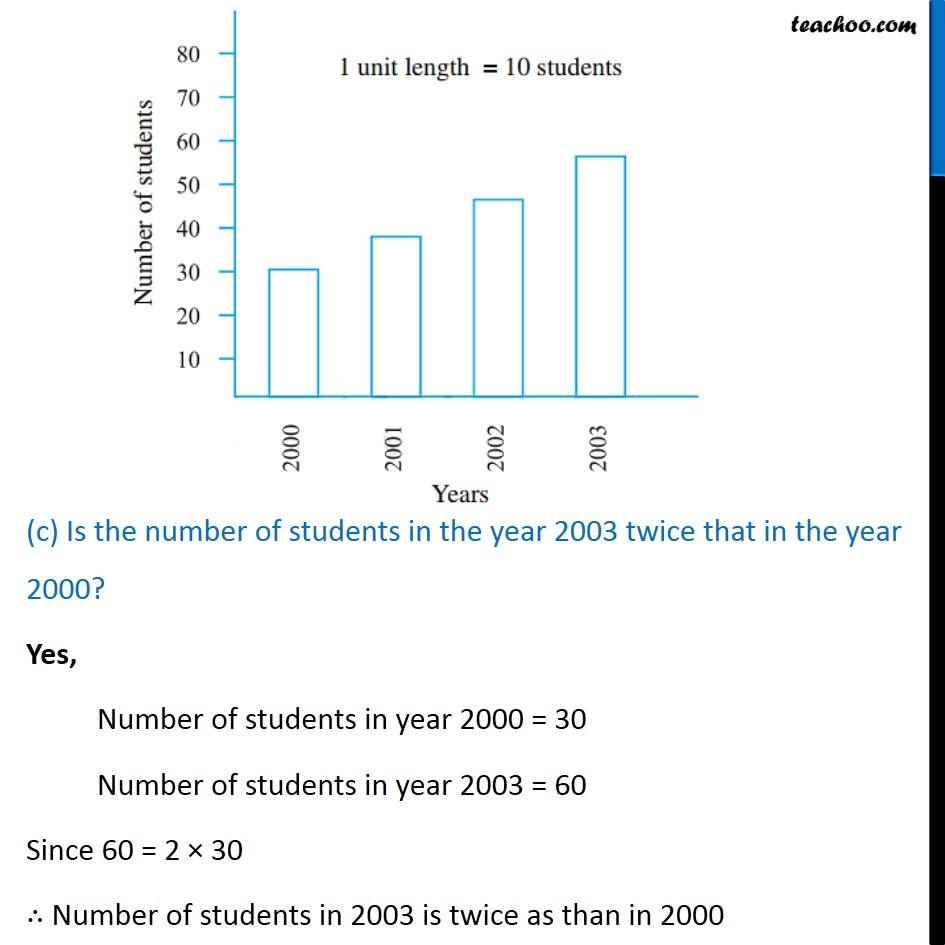Subscribe to our Youtube Channel - https://you.tube/teachoo

1. Chapter 9 Class 6 Data Handling
2. Concept wise
3. Bar Graph

Transcript

Example 9 Read the adjoining bar graph showing the number of students in a particular class of a school. Answer the following questions : What is the scale of this graph? Scale is 1 unit length equals 10 students (b) How many new students are added every year? Number of students in year 2000 = 30 Number of students in year 2001 = 40 Number of students in year 2002 = 50 Number of students in year 2003 = 60 So, 10 new students were added every year (c) Is the number of students in the year 2003 twice that in the year 2000? Yes, Number of students in year 2000 = 30 Number of students in year 2003 = 60 Since 60 = 2 × 30 ∴ Number of students in 2003 is twice as than in 2000

Bar Graph